# let command in Linux with Examples

The let command is used to evaluate arithmetic expressions on shell variables.

Syntax:

`let [expression]`

Options:

• Basic arithmetic operators : The addition(+), subraction(-), multiplication(*), division(/) and modulus(%) operators can be used in the expression with the let command.

Example:

```let "myvar =2" "myvar1=1" "myvar2=myvar1+myvar"; echo \$myvar2
let "myvar =2" "myvar1=1" "myvar2=myvar1-myvar"; echo \$myvar2
let "myvar =2" "myvar1=1" "myvar2=myvar1*myvar"; echo \$myvar2
let "myvar =2" "myvar1=1" "myvar2=myvar1/myvar"; echo \$myvar2
let "myvar =2" "myvar1=1" "myvar2=myvar1%myvar"; echo \$myvar2
```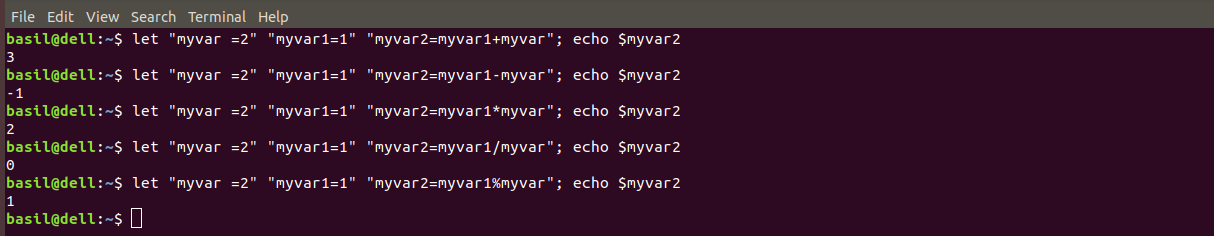• Post-increment(var++) / Post-decrement(var–) operator : This operator is used to interpret the integer value then increase/decrease the integer variable by 1.

Example:

`let "myvar=2" "myvar2=myvar++" ; echo \$myvar \$myvar2`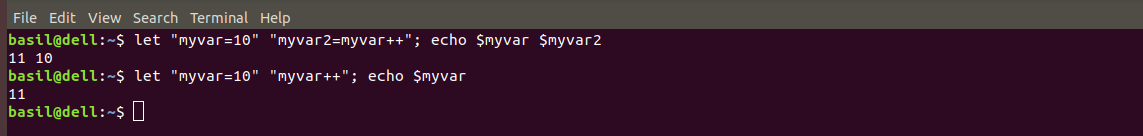In the above example, `myvar2` gets the value of `myvar2` before the increment occurs.

• Pre-increment(++var) / Pre-decrement(–var) operator : This operator increases/decreases the integer value by 1 and then interpret the integer variable.

Example:

`let "myvar=10" "myvar2=--myvar"; echo \$myvar \$myvar2`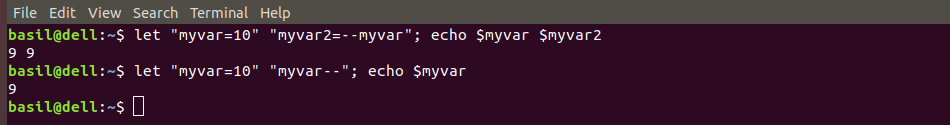In the above example, the change in value occurs first then the value gets stored in `myvar2`.

• Unary plus(+exp) / Unary minus(-exp) : This operator is used to multiply a given expression by 1 or -1.

Example: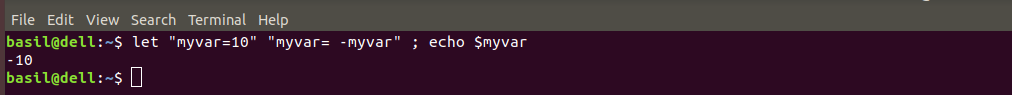In the above example, The value of `myvar` changes from positive to negative with unary minus operator.

• Bit-wise negation(~) : This operator is used to negate every bit of the integer value i.e., turns 0 to 1 and 1 to 0.

Example:

`let "myvar=0" "myvar= ~myvar"; echo \$myvar`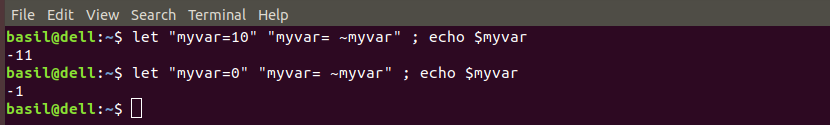In the above example, The value `myvar` is ‘0000…00’ in binary while the negation is ‘1111…11’ which is the 2’s complement value of -1.

• Exponent(**) operator : This operator is used to raise one quantity to the power of another.

Example:

`let "myvar= 5 ** 2" ; echo \$myvar`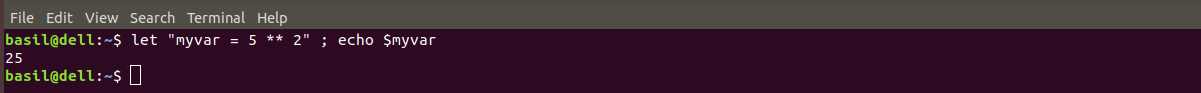• Bitwise shift left / Bitwise shift right : This operator is used to shift the order of the bits either to the left or right.

Example:

`let "myvar = 5 << 2"; echo \$myvar`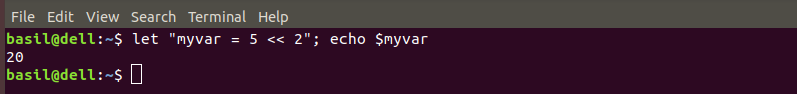• Bitwise AND operator : This operator does a bitwise comparison between two bits and returns 1 if both are 1 else returns 0.

Example:

`let "myvar=5" "myvar2=4" "myvar3 = myvar & myvar2" ; echo \$myvar3`• Bitwise OR operator : This operator does a bitwise comparison between two bits and returns 1 if atleast one the bits is 1, else returns 0.

Example:

`let "myvar=7" "myvar2=4" "myvar3= myvar | myvar2" ; echo \$myvar3`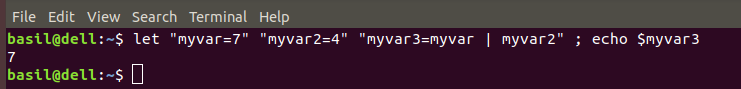• Bitwise XOR operator : This operator does a bitwise comparison between two bits and returns 0 if they are alike, else returns 1.

Example:

`let "myvar=7" "myvar2=4" "myvar3= myvar ^ myvar2" ; echo \$myvar3`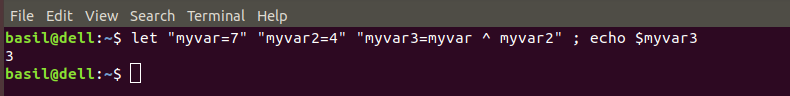My Personal Notes arrow_drop_upCheck out this Author's contributed articles.

If you like GeeksforGeeks and would like to contribute, you can also write an article using contribute.geeksforgeeks.org or mail your article to contribute@geeksforgeeks.org. See your article appearing on the GeeksforGeeks main page and help other Geeks.

Please Improve this article if you find anything incorrect by clicking on the "Improve Article" button below.

Improved By : Akanksha_Rai

Article Tags :

Be the First to upvote.

Please write to us at contribute@geeksforgeeks.org to report any issue with the above content.